# Electron Self Energy Corrections

If one calculates the energy of a point charge using classical electromagnetism, the result is infinite, yet as far as we know, the electron is point charge. One can calculate the energy needed to assemble an electron due, essentially, to the interaction of the electron with its own field. A uniform charge distribution with the classical radius of an electron, would have an energy of the order of. Experiments have probed the electron's charge distribution and found that it is consistent with a point charge down to distances much smaller than the classical radius. Beyond classical calculations, the self energy of the electron calculated in the quantum theory of Dirac is still infinite but the divergences are less severe.

At this point we must take the unpleasant position that this (constant) infinite energy should just be subtracted when we consider the overall zero of energy (as we did for the field energy in the vacuum). Electrons exist and don't carry infinite amount of energy baggage so we just subtract off the infinite constant. Nevertheless, we will find that the electron's self energy may change when it is a bound state and that we should account for this change in our energy level calculations. This calculation will also give us the opportunity to understand resonant behavior in scattering.

We can calculate the lowest order self energy corrections represented by the two Feynman diagrams below.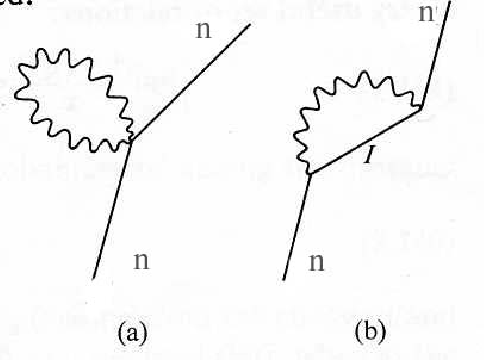In these, a photon is emitted then reabsorbed. As we now know, both of these amplitudes are of order. The first one comes from theterm in which the number of photons changes by zero or two and the second comes from the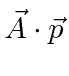term in second order time dependent perturbation theory. A calculation of the first diagram will give the same result for a free electron and a bound electron, while the second diagram will give different results because the intermediate states are different if an electron is bound than they are if it is free. We will therefore compute the amplitude from the second diagram.This contains a term causing absorption of a photon and another term causing emission. We separate the terms for absorption and emission and pull out the time dependence.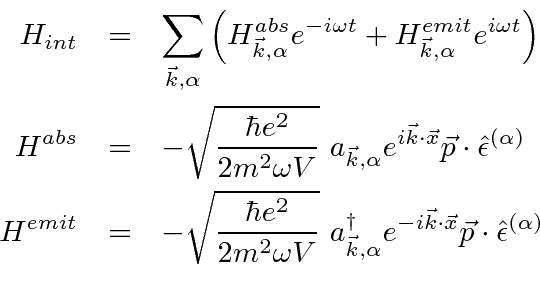The initial and final state is the same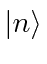, and second order perturbation theory will involve a sum over intermediate atomic states,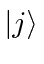and photon states. We will use the matrix elements of the interaction Hamiltonian between those states.We have dropped the subscript on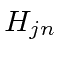specifying the photon emitted or absorbed leaving a reminder in the sum. Recall from earlier calculations that the creation and annihilation operators just give a factor of 1 when a photon is emitted or absorbed.

From time dependent perturbation theory, the rate of change of the amplitude to be in a state is given by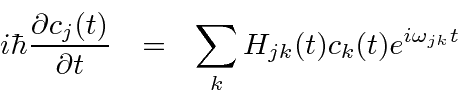In this case, we want to use the equations for the the state we are studying,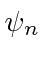, and all intermediate states,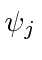plus a photon. Transitions can be made by emitting a photon fromto an intermdiate state and transitions can be made back to the statefrom any intermediate state. We neglect transitions from one intermediate state to another as they are higher order. (The diagram is emit a photon fromthen reabsorb it.)

The differential equations for the amplitudes are then.In the equations for, we explicitly account for the fact that an intermediate state can make a transition back to the initial state. Transitions through another intermediate state would be higher order and thus should be neglected. Note that the matrix elements for the transitions to and from the initial state are closely related. We also include the effect that the initial state can become depleted as intermediate states are populated by using(instead of 1) in the equation for. Note also that all the photon states will make nonzero contributions to the sum.

Our task is to solve these coupled equations. Previously, we did this by integration, but needed the assumption that the amplitude to be in the initial state was 1.

Since we are attempting to calculate an energy shift, let us make that assumption and plug it into the equations to verify the solution.will be a complex number, the real part of which represents an energy shift, and the imaginary part of which represents the lifetime (and energy width) of the state.Substitute this back into the differential equation forto verify the solution and to find out whatis. Note that the double sum over photons reduces to a single sum because we must absorb the same type of photon that was emitted. (We have not explicitly carried along the photon state for economy.)Since this a calculation to orderand the interaction Hamiltonian squared contains a factor ofwe should drop the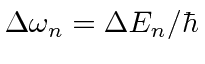s from the right hand side of this equation.We have a solution to the coupled differential equations to order. We should letsince the self energy is not a time dependent thing, however, the result oscillates as a function of time. This has been the case for many of our important delta functions, like the dot product of states with definite momentum. Let us analyze this self energy expression for large time.

We have something of the formIf we think ofas a complex number, our integral goes along the real axis. In the upper half plane, just above the real axis,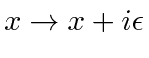, the function goes to zero at infinity. In the lower half plane it blows up at infinity and on the axis, its not well defined. We will calculate our result in the upper half plane and take the limit as we approach the real axis.This is well behaved everywhere except at. The second term goes tothere. A little further analysis could show that the second term is a delta function.Recalling that, the real part ofcorresponds to an energy shift in the stateand the imaginary part corresponds to a width.All photon energies contribute to the real part. Only photons that satisfy the delta function constraint contribute to the imaginary part. Moreover, there will only be an imaginary part if there is a lower energy state into which the state in question can decay. We can relate this width to those we previously calculated.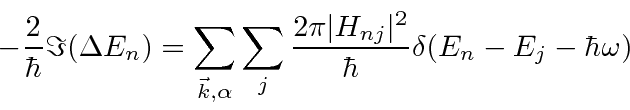The right hand side of this equation is just what we previously derived for the decay rate of state, summed over all final states.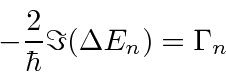The time dependence of the wavefunction for the stateis modified by the self energy correction.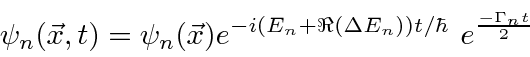This also gives us the exponential decay behavior that we expect, keeping resonant scattering cross sections from going to infinity. So, the width just goes into the time dependence as expected and we don't have to worry about it anymore. We can now concentrate on the energy shift due to the real part of.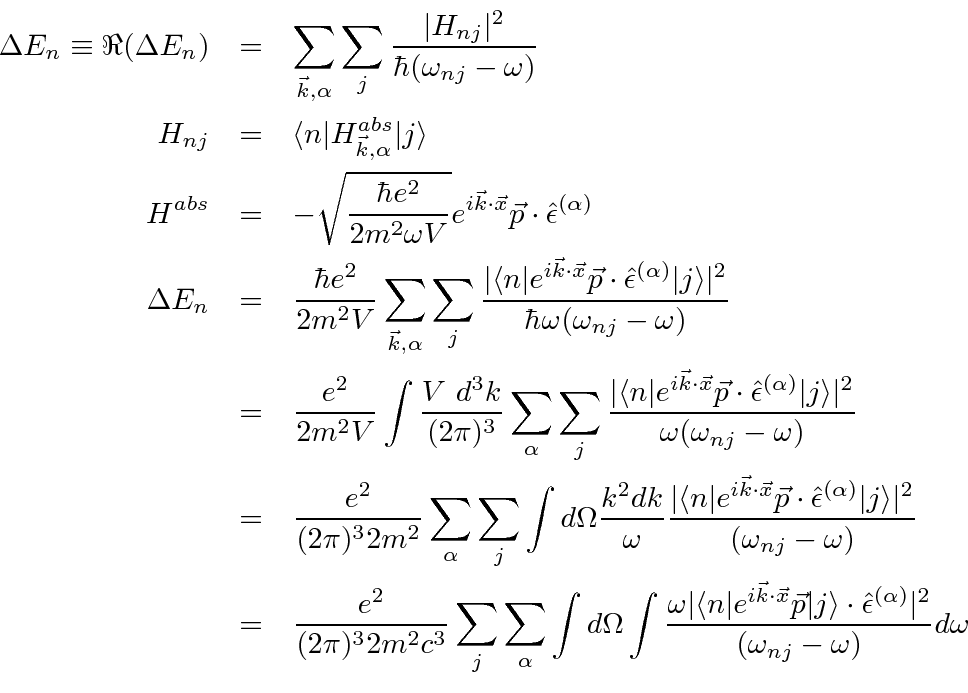In our calculation of the total decay rate summed over polarization and integrated over photon direction we computed the cosine of the angle between each polarization vector and the (vector) matrix element. Summing these two and integrating over photon direction we got a factor ofand the polarization is eliminated from the matrix element. The same calculation applies here.Note that we wish to use the electric dipole approximation which is not valid for large. It is valid up to about 2000 eV so we wish to cut off the calculation around there. While this calculation clearly diverges, things are less clear here because of the eventually rapid oscillation of theterm in the integrand as the E1 approximation fails. Nevertheless, the largest differences in corrections between free electrons and bound electrons occur in the region in which the E1 approximation is valid. For now we will just use it and assume the cut-off is low enough.

It is the difference between the bound electron's self energy and that for a free electron in which we are interested. Therefore, we will start with the free electron with a definite momentum. The normalized wave function for the free electron is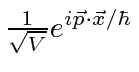.It easy to see that this will go to negative infinity if the limit on the integral is infinite. It is quite reasonable to cut off the integral at some energy beyond which the theory we are using is invalid. Since we are still using non-relativistic quantum mechanics, the cut-off should have. For the E1 approximation, it should be. We will approximatesince the integral is just giving us a number and we are not interested in high accuracy here. We will be more interested in accuracy in the next section when we compute the difference between free electron and bound electron self energy corrections.If we were hoping for little dependence on the cut-off we should be disappointed. This self energy calculated is linear in the cut-off.

For a non-relativistic free electron the energydecreases as the mass of the electron increases, so the negative sign corresponds to a positive shift in the electron's mass, and hence an increase in the real energy of the electron. Later, we will think of this as a renormalization of the electron's mass. The electron starts off with some bare mass. The self-energy due to the interaction of the electron's charge with its own radiation field increases the mass to what is observed.

Note that the correction to the energy is a constant times, like the non-relativistic formula for the kinetic energy.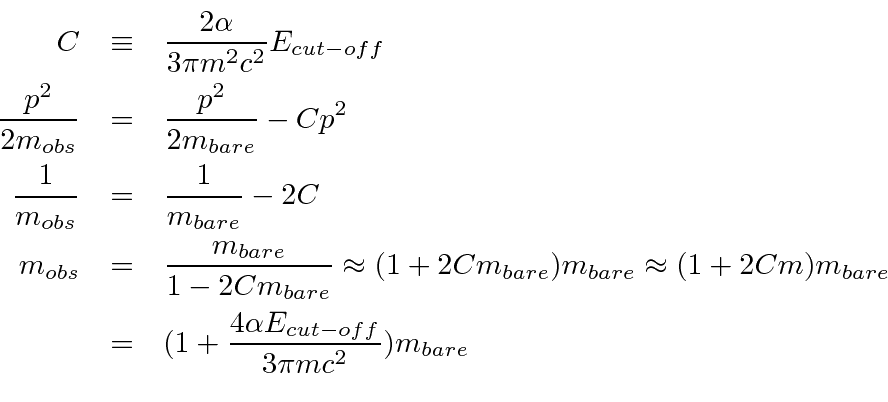If we cut off the integral at, the correction to the mass is only about 0.3%, but if we don't cut off, its infinite. It makes no sense to trust our non-relativistic calculation up to infinite energy, so we must proceed with the cut-off integral.

If we use the Dirac theory, then we will be justified to move the cut-off up to very high energy. It turns out that the relativistic correction diverges logarithmically (instead of linearly) and the difference between bound and free electrons is finite relativistically (while it diverges logarithmically for our non-relativistic calculation).

Note that the self-energy of the free electron depends on the momentum of the electron, so we cannot simply subtract it from our bound state calculation. (What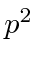would we choose?) Rather me must account for the mass renormalization. We used the observed electron mass in the calculation of the Hydrogen bound state energies. In so doing, we have already included some of the self energy correction and we must not double correct. This is the subtraction we must make.

Its hard to keep all the minus signs straight in this calculation, particularly if we consider the bound and continuum electron states separately. The free particle correction to the electron mass is positive. Because we ignore the rest energy of the electron in our non-relativistic calculations, This makes a negative energy correction to both the bound () and continuum (). Bound states and continuum states have the same fractional change in the energy. We need to add back in a positive term into avoid double counting of the self-energy correction. Since the bound state and continuum state terms have the same fractional change, it is convenient to just usefor all the corrections.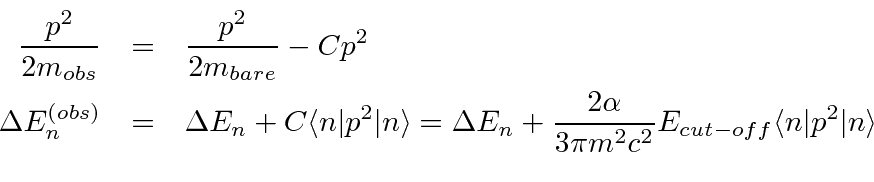Because we are correcting for the mass used to calculate the base energy of the state, our correction is written in terms of the electron's momentum in that state.

Subsections
Jim Branson 2013-04-22